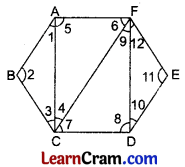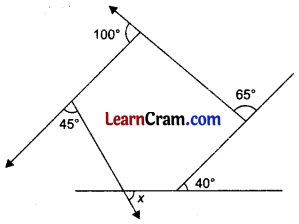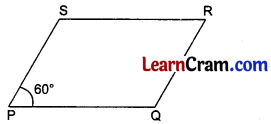# DAV Class 8 Maths Chapter 11 Worksheet 1 Solutions

The DAV Maths Class 8 Solutions and DAV Class 8 Maths Chapter 11 Worksheet 1 Solutions of Understanding Quadrilaterals offer comprehensive answers to textbook questions.

## DAV Class 8 Maths Ch 11 WS 1 Solutions

Question 1.
Find the sum of interior angles of hexagon ABCDEF by dividing it into triangles.Solution:
Given: ABCDEF is a hexagon.
Joining AC, CF, and DF, we get four triangles.In ΔABC, ∠1 + ∠2 + ∠3 = 180° ………(i)
In ΔACF, ∠4 + ∠5 + ∠6 = 180° ………(ii)
In ΔCDF, ∠7 + ∠8 + ∠9 = 180° ……….(iii)
In ΔDEF, ∠10 + ∠11 + ∠12 = 180° ……….(iv)
Adding (i), (ii), (iii) and (iv), we get
∠1 + ∠2 + ∠3 + ∠4 + ∠5 + ∠6 + ∠7 + ∠8 + ∠9 + ∠10 + ∠11 + ∠12 = 180° + 180° + 180° + 180°
⇒ (∠1 + ∠5) + ∠2 + (∠3 + ∠4 + ∠7) + (∠6 + ∠9 + ∠12) + (∠8 + ∠10) + ∠11 = 720°
⇒ ∠A + ∠B + ∠C + ∠F + ∠D + ∠E = 720°
⇒ ∠A + ∠B + ∠C + ∠D + ∠E + ∠F = 720°Question 2.
Find the sum of interior angles of a polygon of a given number of sides by using the formula (n – 2) × 180°.
(i) 12 sides
(ii) 9 sides
(iii) 22 sides
Solution:
(i) Given: n = 12
Then, the sum of interior angles of a polygon having 12 sides = (12 – 2) × 180°
= 10 × 180°
= 1800°

(ii) Given: n = 9
Then, the sum of the interior angles of a polygon having 9 sides = (9 – 2) × 180°
= 7 × 180°
= 1260°

(iii) Given: n = 22
Then, the sum of the interior angles of a polygon having 22 sides = (22 – 2) × 180°
= 20 × 180°
= 3600°

Question 3.
Find the measure of each angle of a regular octagon.
Solution:
Number of sides of a regular octagon, n = 8
Measure of each angle of a regular octagon = $$\frac{(n-2) \times 180^{\circ}}{n}$$
= $$\frac{(8-2) \times 180^{\circ}}{8}$$
= $$\frac{6 \times 180^{\circ}}{8}$$
= 3 × 45°
= 135°Question 4.
Four angles of a pentagon are 100°, 175°, 85° and 75°. Find the fifth angle.
Solution:
The number of angles of a pentagon = 5
Sum of five angles = (5 – 2) × 180°
= 3 × 180°
= 540°
Let the fifth angle of the pentagon be x.
Then, 100° + 175° + 85° + 75° + x = 540°
⇒ 435° + x = 540°
⇒ x = 540° – 435°
⇒ x = 105°

Question 5.
Find the measure of x in the given figure.Solution:
We know that the sum of measures of exterior angles of a pentagon is 360°.
∴ 100° + 45° + x + 40° + 65° = 360°
⇒ x + 250° = 360°
⇒ x = 360° – 250°
⇒ x = 110°### DAV Class 8 Maths Chapter 11 Worksheet 1 Notes

• Quadrilateral is a polygon enclosed by four sides.
• The sum of all the interior angles of a quadrilateral is 360°.
• The sum of all interior angles of a pentagon is 540°.
• The sum of all exterior angles of any polygon is 360°.
• Trapezium is a quadrilateral in which one pair of opposite sides is parallel.
• Parallelogram is a quadrilateral in which (i) opposite sides are equal and parallel to each other (ii)
• opposite angles are equal to each other.
• Diagonals of a parallelogram bisect each other.
• Rhombus is a quadrilateral whose all sides are equal and its diagonals bisect each other at right angle.
• Rectangle is a quadrilateral whose each angle is 90°, opposite sides are equal and
• parallel to each other. Its diagonals are equal and bisect each other.
• Square is a quadrilateral whose all sides are equal. Each angle is 90°. Its diagonals are equal and bisect each other at right angles.Example 1.
In the given figure find the measure of ∠x.Solution:
Sum of all the exterior angles of a polygon is 360°.
∴ ∠x + ∠50° + ∠70° + ∠130° = 360°
⇒ ∠x + 250° = 360°
⇒ ∠x = 360 – 250
∴ ∠x = 110°.

Example 2.
PQRS is a parallelogram in which ¿P = 60°, find the remaining angles of the parallelogram.Solution:
∠P + ∠Q = 180° [Sum of interior angles]
⇒ 60°+ ∠Q = 180°
∴∠Q = 180° – 60° = 120°
∠P = ∠R
[Opposite angles of a parallelogram]
∴ ∠R = 60°
Similarly ∠Q = ∠S
∴ ∠S = 120°.

Example 3.
The adjacent sides of a parallelogram are in the ratio 3 : 4. If its perimeter is 140 cm, find the sides.
Solution:
Let the two adjacent sides be 3x and 4x cm.
∴ Perimeter = 2 (3x + 4x) = 14x cm
14x = 140
∴ x = 10
Hence the sides are 3 × 10 = 30 cm and 4 × 10 = 40 cm.Example 4.
The diagonals of a rhombus are in the ratio 3 : 4. If its perimeter is 40 cm, find the length of sides and the diagonals of the rhombus.Solution:
Perimeter = 40 cm
4 × Side = 40 cm
∴ Side = 10 cm
AC : BD = 4 : 3
As the diagonals of the rhombus bisect at 90°,
∴ In rt.ΔAOB
OA = $$\frac{1}{2}$$ AC
= $$\frac{1}{2}$$ × 4x = 2x

OB = $$\frac{1}{2}$$ BD
= $$\frac{1}{2}$$ × 3x
= $$\frac{3}{2}$$ x

From Pythagorous Theorem,
OA2 + OB2 = AB2
(2x)2 + ($$\frac{3}{2}$$x)2 = (10)2
4x2 + $$\frac{9 x^2}{4}$$ = 100
16x2 + 9x2 = 400
25x2 = 400
x2 = $$\frac{400}{25}$$ = 16
∴ x = 4.
Hence AB = BC = CD = DA = 10 cm
AC = 4 × 4 = 16 cm
and BD = 3 × 4 = 12 cm.